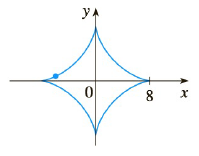Chapter 2.6, Problem 30E

Chapter
Section
Textbook Problem

# Use implicit differentiation to find an equation of the tangent line to the curve at the given point. x 2 / 3 + y 2 / 3 = 4 , ( − 3 3 , 1 ) (asteroid)To determine

To find:

Equation of tangent line to the curve at a given point by using implicit differentiation.

Explanation

1) Concept:

Slope of tangent line is derivative of curve at that point. Find the derivative by using implicit differentiation and substitute given point in it. This will be the slope of tangent line. Use the equation of point slope form for the given point and the calculated slope to find the equation of tangent line.

2) Formula:

i. The Power rule

ddxxn=nxn-1

3) Given:

x2/3+y2/3=4

4) Calculation:

Curve x2/3+y2/3=4, point (-33,1)

Differentiate with respect to x

ddxx2/3+y2/3=0

ddxx2/3+ddxy2/3=0

23x1/3+23y1/3dydx=0

1x1/3+1y1/3dydx=0

1y1/3dydx=-1x1/3

d

### Still sussing out bartleby?

Check out a sample textbook solution.

See a sample solution

#### The Solution to Your Study Problems

Bartleby provides explanations to thousands of textbook problems written by our experts, many with advanced degrees!

Get Started

#### In Problems 15-28, find the general solution to the given differential equation.

Mathematical Applications for the Management, Life, and Social Sciences

#### The solution to xdydx2y=x3 is: a) y=ex3+Cx2 b) y=ex3+x2+C c) y=x3+x2+C d) y=x3+Cx2

Study Guide for Stewart's Single Variable Calculus: Early Transcendentals, 8th

#### Define the concept of internal validity and a threat to internal validity.

Research Methods for the Behavioral Sciences (MindTap Course List)